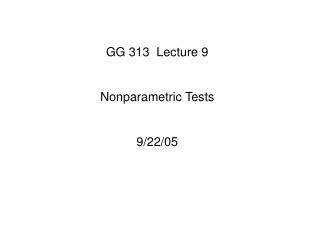# GG 313 Lecture 9 Nonparametric Tests 9/22/05 - PowerPoint PPT PresentationDownload PresentationGG 313 Lecture 9 Nonparametric Tests 9/22/05

GG 313 Lecture 9 Nonparametric Tests 9/22/05Download Presentation## GG 313 Lecture 9 Nonparametric Tests 9/22/05

- - - - - - - - - - - - - - - - - - - - - - - - - - - E N D - - - - - - - - - - - - - - - - - - - - - - - - - - -
##### Presentation Transcript

1. GG 313 Lecture 9 Nonparametric Tests 9/22/05

2. If we cannot assume that our data are at least approximately normally distributed - because there are a small number of elements in the sample, the distribution is unknown, or the data are ordinal (can only be ranked), then we must use non-parametric tests to evaluated hypotheses. These tests do not use the standard statistics of mean and standard deviation. SIGN TEST for one-sample mean or median: Robust replacement for 1-sampe t-test. Requires that the sampled distribution is continuous and symmetrical. If the population is not symmetrical, the test applies to the median rather than the mean.

3. Sign test: We test whether out mean (or median) is statistically indistinguishable from a hypothetical value. The properties of the binomial distribution are invoked. The question asked is: What is the probability of finding x values out of n less than the mean (or median), following directly from eqn. 1.117. EXAMPLE: Random salinity measurements (P.44). We hypothesize that the salinity is less than 98.5, so our null hypothesis is that the salinity = 98.5. We COUNT the number of values that are greater than 98.5, ignore all values that equal 98.5, and find that there are two values greater and one equal out of 15. So we want to know the probability that 2 values or less out of 14 will be less than 98.5.

4. From equation 1.117: In our case, p=the probability that an event will be less than or greater than 98.5 is 1/2, and we want to sum the probabilities for 0, 1, and 2 occurrences of a number less than 98.5: (eqn. 2.31) This says that the probability that the salinity is less than 98.5 is 0.66%. Since this is less than 1%, we can reject Ho.

5. If np and n(1-p) are both > 5, we can use the normal approximation to the binomial distribution, and use the z statistic: Eqn: 2.32 Mann-Whitney Test This is a non-parametric alternative to the 2-sample t-test. Matlab and others know it as the WILCOXON Test. The data from two samples are tested to see if they come from the same population. The two samples are combined, sorted, and then ranked from 1 to n1+n2.

6. If two or more values are the same, they each get the average rank of that group. For example if the 8, and 9th ranked samples each have the same value, they both get ranked 8.5. The expectation is that the values from each sample will be scattered more or less uniformly in the rankings if they come from the same population. After ranking, we split the samples apart again and get the rank sums, W1 and W2 for each sample. The sum of the rank sums is: Eqn: 2.33 This is the sum of integers from 1 to n1+n2.

7. We define the U statistic as: Eqn: 2.34 2.35 U is defined as the smaller of U1 and U2. U varies from 0 to n1*n2, and it is symmetrical about n1*n2/2. Our test is to compare U with the critical U, obtained from a table. (I have not been able to find the equivalent in Matlab). The table is presented on the next page:

8. Example: Grain size of lunar sands. Two samples were taken at different parts of the moon. Do they come from different populations? Move to EXCEL demonstration.

9. Kolmogorov-Smirnov Test This test is used to test goodness of fit or shape and can be used instead of the chi^2 test. With this test, you do not have to bin the data. It simply needs the maximum difference between two cumulative distribution curves. Steps: • Sort data from smallest to largest. • Convert the data distribution to a cumulative distribution S(x). S(x) gives the fraction of the data points that are to the left of x. The smallest x is 0 and the largest is 1. •  Plot the cumulative distribution along with the comparison distribution. •Find the maximum absolute difference.

10. Using Matlab function “kstest” or a table, we find the K-S value for our alpha and n, and compare with the maximum difference we observed. Non-parametric Correlation Also called rank correlation or Spearman’s rank correlation, rs. • Rank the x and y values seperately.• Find the difference in rank between xi and yi pairs • evaluate rs: Eqn: 2.41

11. If the null hypothesis (no correlation) is true, the distribution of rs has zero mean and a standard deviation of (n-1)^-.5. Since this is a normal distribution we can use z- statistics: Eqn 2.41 Example: Using the dice throws from earlier: (page 48):